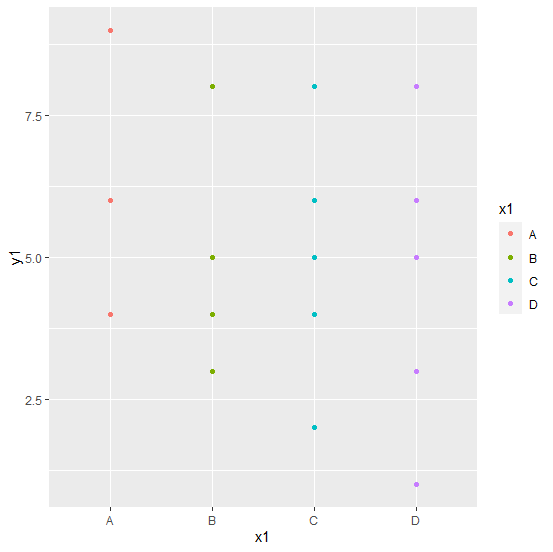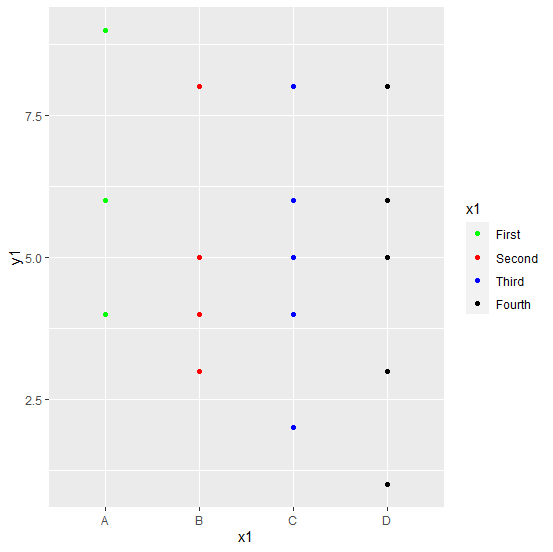# How to change legend values in a bar plot created by using ggplot2 in R?

How to change legend values in a bar plot created by using ggplot2 in R?

By default, the legend values are taken as the different levels of the categorical variable for which a bar plot is created using ggplot2 package and if we want to change those values then scale_color_manual function of the ggplot2 package can be used where we need to pass the values for color and labels for legend values.

## Example

Consider the below data frame:

Live Demo

> set.seed(1214)
> x1<-sample(LETTERS[1:4],20,replace=TRUE)
> y1<-rpois(20,5)
> df1<-data.frame(x1,y1)
> df1

## Output

  x1 y1
1  B  4
2  B  5
3  C  5
4  C  8
5  A  4
6  B  8
7  C  6
8  C  5
9  C  2
10 A  6
11 A  9
12 D  5
13 D  8
14 D  8
15 B  3
16 D  1
17 D  3
18 D  6
19 D  3
20 C  4

Loading ggplot2 package and creating bar plot for x1 with y1 as frequencies:

> library(ggplot2)
> ggplot(df1,aes(x1,y1,color=x1))+geom_point()Creating the bar plot with different legend values:

> ggplot(df1,aes(x1,y1,color=x1))+geom_point()+scale_color_manual(values=c("green","red","blue","black"),labels=c("First","Second","Third","Fourth"))

## Output: